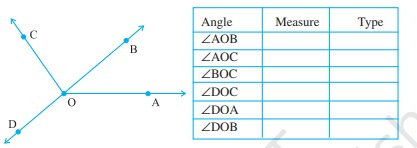" ">

# Measure and classify each angle :"

To do:

We have to measure and classify each angle.

Solution:

Using Protractor,

The measure of $\angle AOB$ is $40^o$ and it is an acute angle.

The measure of $\angle AOC$ is $125^o$ and it is an obtuse angle.

The measure of $\angle BOC$ is $85^o$ and it is an acute angle.

The measure of $\angle DOC$ is $95^o$ and it is an obtuse angle.

The measure of $\angle DOA$ is $140^o$ and it is an obtuse angle.

The measure of $\angle DOB$ is $180^o$ and it is a straight angle.

Updated on: 10-Oct-2022

26 Views